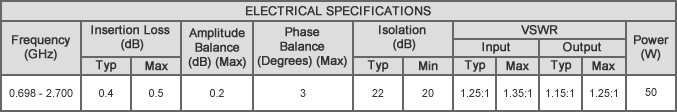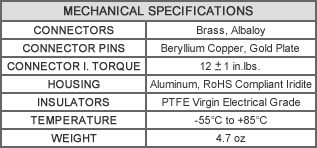# P2N-1.700V, N-Female, 0.698-2.7 GHz

Original price - Original price
Original price
-
Current price
SKU P2N-1.700V

Description:
MECA's 2-way, P2N-1.700V, Tee N-Female power divider/combiner with an average power rating of 50 watts and frequency of 0.698-2.7 GHz.Notes:
1. All units rated for maximum input power as a divider or balanced combiner with load
VSWR of 1.20:1 or better. To calculate the combining rated power the following formula is used:
(rated input power of divider * 5%) / "N" # of input channels = max input at each port for combining.
2. All output/power combiner ports are in-phase (0° difference).
3. 50 ohm nominal impedance standard.
4. Operating temperature from -55°C to +85°C.
5. See application note MAP-801